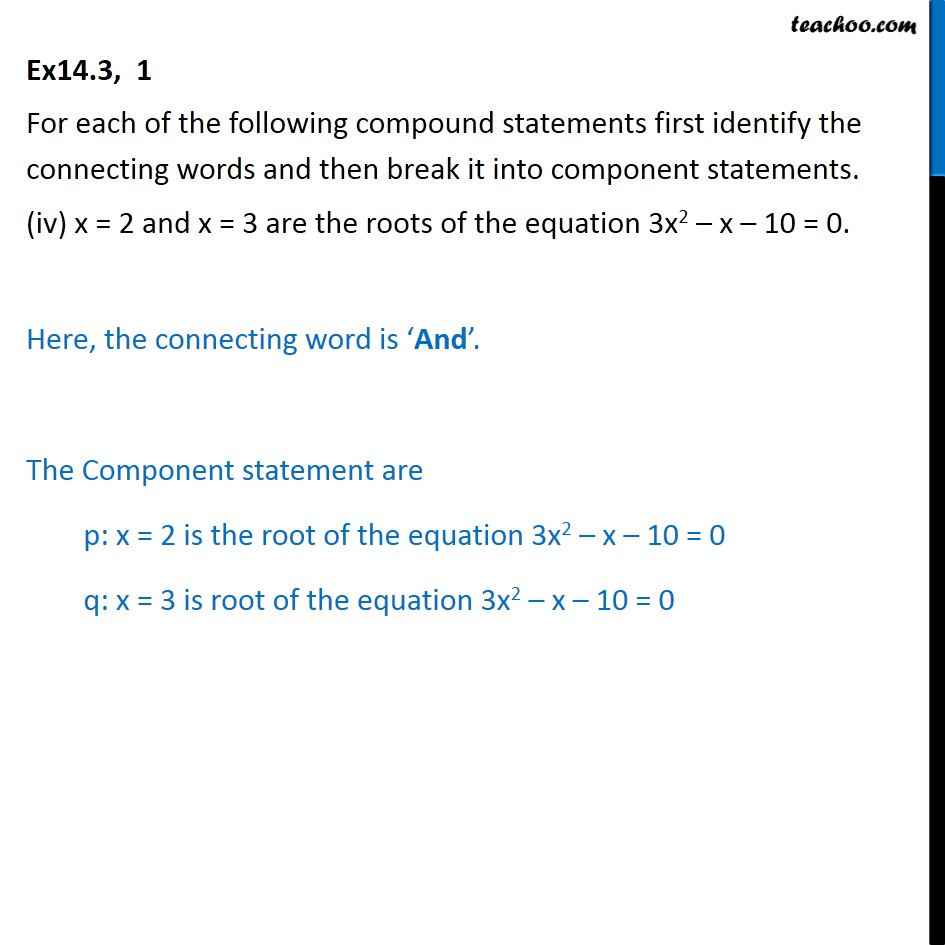Compound Statements

Mathematical Reasoning
Serial order wiseLearn in your speed, with individual attention - Teachoo Maths 1-on-1 Class

### Transcript

Ex14.3, 1 For each of the following compound statements first identify the connecting words and then break it into component statements. (iv) x = 2 and x = 3 are the roots of the equation 3x2 – x – 10 = 0. Here, the connecting word is ‘And’. The Component statement are p: x = 2 is the root of the equation 3x2 – x – 10 = 0 q: x = 3 is root of the equation 3x2 – x – 10 = 0